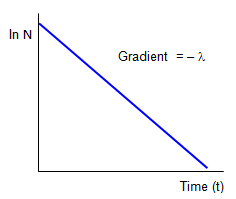# Mathematical consideration of radioactive decay

Consider the number of nuclei (dN) decaying in a short time dt.
dN is proportional to:-
N - the number of radioactive nuclei present at that moment
dt - the time over which the measurement is made
the element, represented by a constant (λ) called the disintegration (or decay) constant.
So:

dN = - λNdt Activity: dN/dt = - λN

the minus sign is there because the number of radioactive nuclei decreases as time increases.
The quantity dN/dt is the rate of decay of the source or the activity of the source and is the number of disintegrations per second.

This is measured in units called Becquerels (Bq) where 1 Bq = 1 disintegration per second.
A larger and more traditional unit is the Curie (Ci) 1 Ci = 3.7 x1010 Bq.

The disintegration constant or decay constant (λ) can be defined as the probability of a nucleon decaying in the next second.

The number of nuclei in a sample can be related to the mass of the source (m) using the molar mass (M) and Avogadro's number (L) by the formula:-

m = MN/L

and we can use it to find out the mass of a given source if we know its activity.

Example problem
A school has a radium 226 source with an activity of 5 mCi (5x10-6x3.7x1010 Bq).
What is the mass of the source?
Avogadro constant (L) = 6.02x1023) and the disintegration constant for radium 226 is 1.35x10-11 s-1
(see later for an explanation of this term)
Using the formula dN/dt = -λN = -λ(m/M)L
so 5x10-6x3.7x1010 = 1.35x10-11(m/226)6.02x1023

Therefore m = (5x10-6x3.7x1010x 226)/ 1.35x10-11 x 6.02x1023 = 5.14x10-6 grams = 5.14 μg

Returning to the formula
dN/dt = -λN and rearranging gives:
dN/N = -λdt which when integrated between the limits N = No and N = N for the number of nuclei at time 0 and t gives:ln No – ln N = λt

If we plot ln(N) against t we have a straight line graph with gradient -λ) and an intercept on the ln(N) axis of ln(No). It is this sort of graph that would be most helpful in finding the half life (T) by measuring the gradient and then using the relation between the half-life and the disintegration constant (see below).

Returning to the equation and taking antilogs of both sides gives:

Radioactive decay law: N = Noe-λt

A graph of N against t would give an exponential decay graph, and if background radiation were ignored the line would tend towards N = 0 as time goes by. Since N is directly proportional to the activity (A) and the mass (m) of the sample we have three alternative forms of this formula.
It can be expressed as:

(Number of nuclei) N = Noe-λt     (Activity) A = Aoe-λt      (Mass) m = moe-λt

### Half life and the radioactive decay constant

We can now get a much better idea of the meaning of not only the half life (T) but also of the decay constant (λ).
When N = No/2 the number of radioactive nuclei will have halved and so one half life will have passed.
Therefore when t = T N = No/2 = Noe-λT and so 1/2 = e-λT .
Taking the inverse gives 2 = eλT and so:

ln(2) = 0.693 = λT and so
Decay constant (λ) = 0.693/T

Example problem
The decay constant (λ) of a particular isotope (radon 220) is 1.33x10-2 s-1.
How long will it take for the activity of a sample of this isotope to decay to one eighth of its original value?
Half life = 0.693/λ = 0.693/1.33x10-2 = 52 s.
Number of half lives required to reduce the activity to one eighth = 3
Therefore time needed = 3 x 52 = 156 s = 2m 36s

### Half lives and decay constants

The following table gives some values of half lives and decay constants. Notice that short half lives go with large decay constants - a radioactive material with a short half life will obviously lose its radioactivity rapidly.

 Isotope Half life Decay constant (s-1) Uranium 238 4.5x109 years 5.0x10-18 Plutonium 239 2.4x104 years 9.2x10-13 Carbon 14 5570 years 3.9x10-12 Radium 226 1622 years 1.35x10-11 Free neutron 239 15 minutes 1.1x10-3 Radon 220 52 seconds 1.33x10-2 Lithium 8 0.84 seconds 0.825 Bismuth 214 1.6x10-4 seconds 4.33x103 Lithium 8 6x10-20 seconds 1.2x1019

### Proof of A = Ao/2n

Start with the standard radioactive decay law and take logs to the base e:

A = Aoe-λt
ln A = ln o-λt = ln Ao – ln2(t/T) where T is the half life.

Therefore: ln A = ln(Ao/2n) where n = t/T and so A = Ao/2n

Example problems
1. A sample of material is found to contain 2 g of the isotope gold 199.
How much will remain 10 days later?
The half life of gold 199 is 3.15 days and so the decay constant is therefore 0.693/3.15x86400 = 2.55x10-6 s-1. However since the half life and the time over which the decay takes place are both given in days we do not need to change both into seconds.
Using the formula:- m = moe-λt we have m = 2xe-(0.693/3.15)10 = 0.22 g

2. A sample of carbon 14 has an activity of 2.5 Bq when corrected for background radiation.
If the initial count rate of a sample of the same mass was 3.7 Bq how old is the sample?
(Half life of carbon 14 = 5570 years).
Using the formula A = Aoe-λt we have 2.5 = 3.7e-(0.693/5570)t where t is in years.
Taking logs gives: ln(2.5/3.7) = -(0.693/5570)t this gives t = 3151 years.

A VERSION IN WORD IS AVAILABLE ON THE SCHOOLPHYSICS USB

© Keith Gibbs